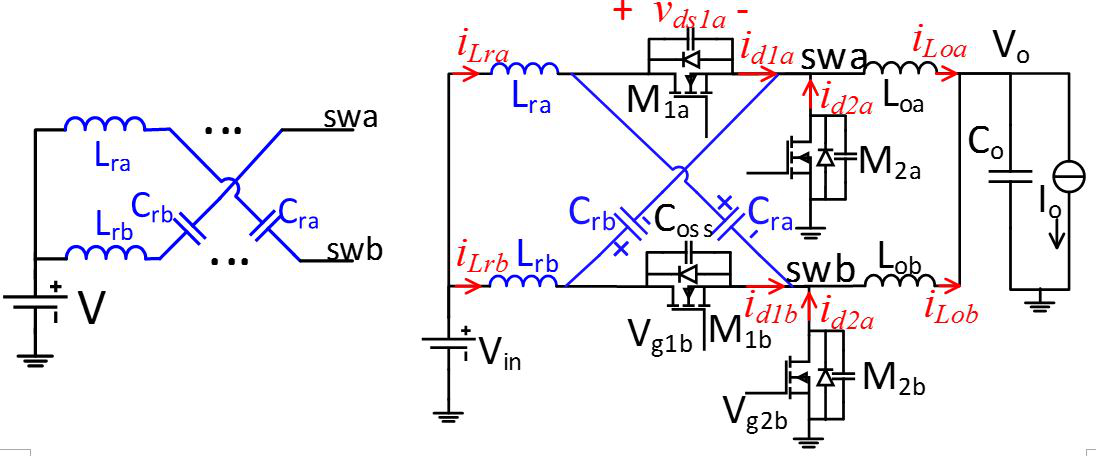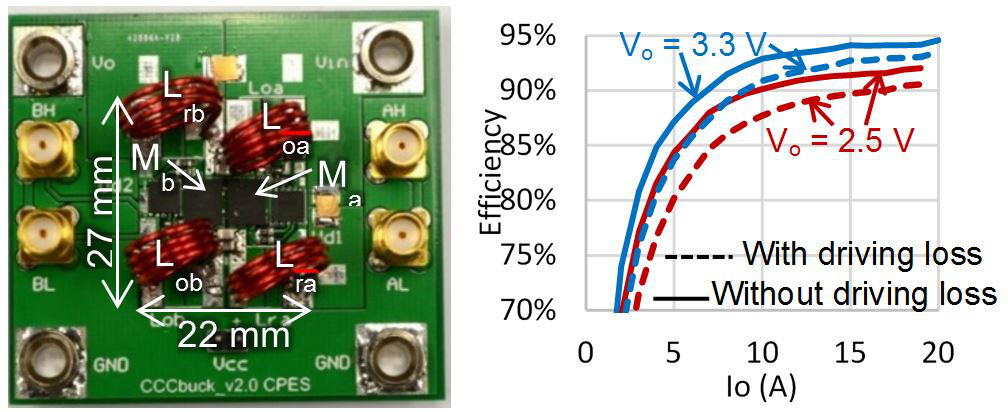LIBRARY

# Resonant Cross-Commutated DC-DC ConverterFig. 1. Resonant cross-commutated cell applied in two-phase buck converter.
The resonant current generated in one phase of two interleaved buck converters is injected into the switched node of the other phase to turn on the active switch at zero voltage over wide load range, and to turn off the synchronous switch at near-zero current at the rated load. A general two-phase resonant cross-commutated (rcc) cell is illustrated in Fig. 1 (a). The current in the resonant tank of phase b is injected into the switched node swa of phase a. This idea is applied to an interleaved two-phase buck converter with output voltage regulated by extended duty ratio. Parametric analysis is performed to study the impact of the resonant components on voltage and current stresses. The rccBuck converter is exemplified in Fig. 1 (b).

The operating principles and stresses are verified by an example with Vin = 12 V, Vo = 3.3 V, IoMax = 20 A, Ipp_Lo = 30% IoMax, VdsMax = 1.6 Vin, and fs = 2 MHz. Resonant cross-commutation has been proven effective (by simulation and experiment) in turning on the active switch at zero voltage over wide load range, and turning off the synchronous switch below 10 percent of IoMax at rated load, and below 25 percent of IoMax at half load. A 12 V3 V rccBuck converter switched at 2 MHz was designed and demonstrated to have peak efficiency of 93.5 percent at 20 A. The root mean square (rms) current in the resonant inductors is kept below 0.4 Io at half load by utilizing resonance between Lr and Cr. The duty ratio is extended and current stress for active metal-oxide semiconductor field-effect transistors (MOSFETs) is reduced compared with critical-mode buck converters. The concept of resonant cross-commutation can be extended to other topologies, such as boost converters, buck-boost converter, among others.Fig. 2. (a) Prototype of rccBuck converter with Vin = 12 V, Io = 20 A, and fs = 2 MHz; (b) efficiencies versus load current.